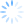Sometimes it's particularly important to get accurate distance information between addresses, and when managing large address lists, the fastest and most accurate approach is to first geocode the locations (get their latitude and longitude) and then use this information as the basis of the distance calculations.  CDXZipStream does this particularly well with a combination of two functions, CDXLocateMP and CDXDistance2WP.  We're going to review step-by-step how you can use these two functions within Microsoft Excel, to filter an address list based on the calculated straight-line (as the crow flies) distance to a target address.

Let's say you have a long customer address list and would like to determine which ones are closest to a specific store location.  In many cases, especially in sparsely populated areas where zip codes may cover hundreds of square miles, it's not appropriate to use the centroid location of the zip codes as the basis of the distance calculations, the approach used in many zip code-to-zip code calculators.  To get accurate address-to-address distances, first geocode all addresses using CDXLocateMP.  The input box would look like this, where Excel cell references are used as the input for each address.This is a multi-line address where the street, city, state and zip code are listed in cells A2 through D2.  We also request that latitude is returned to the worksheet cell where the cursor is pointed, and here is the result returned to cell E2:We repeat the process to get the longitude returned to cell F2, by simply specifying longitude as the returned data.  Now all we have to do is copy and paste the formulas in these cells to the rest of the list, and all customer addresses are now geocoded.

After also geocoding the location of the targeted store, we're now ready to calculate the actual distances to the store based on latitude and longitude, using the function CDXDistance2WP.  Since this function uses a straightforward mathematical formula, the calculations are very fast.  Here's an example of the CDXDistance2WP input:We use cell addresses for the data, in this case the geocoded "waypoint".  For the target location, we also specify an unchanging cell location using the dollar sign (\$) before the column letters and row numbers.

To get distance data for the entire list, we again just copy the resulting equation from the first row of data all along the list of geocoded points.  To see a short tutorial showing the use of both CDXLocate and CDXDistance2WP functions, please view the following video:

TheYouTube version is here: Geocoder in Excel

Now use Excel's sort capabability to sort the distances, or use the autofilter function for more advanced features.  Autofilter can easily show you all the distances that fall within a radius.  Let's say we want to narrow the list of addresses to those within a 50 mile radius of the target address.  In Excel 2007 and 2010, follow this procedure:

1.Use your cursor to select all the data (addresses and distances) you wish to filter.

2.From the Data tab, select Filter.

3.You should now see drop-down buttons at the top of each column of data.  (You should be using column headings here so the buttons don't cover the first row of data.) Click on the drop-down button in the column of distance data.

4.Click on "Number Filters"

5.Select "Less Than or Equal to"

6.Enter the value "50" in the box to the right of "Less than or Equal to"

All rows that do not meet the 50 mile or less requirement are now hidden.  You can now copy and paste the filtered data to a new workbook to save it.